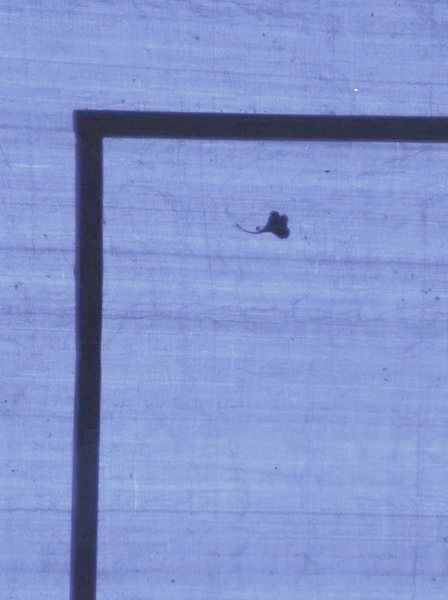explorations, interests & opinions of a mind

# CLRS - problem 2.3-7

Solution to problem 2.3-7, Introduction to Algorithms, CLRS, 2nd ed.

## Problem:

We are given a set $S$ of $n$ integers, and another integer $x$. And we need to find out if there exists two integers in the set $S$ such that there sum is $x$.

## Approach:

The straight forward answer is to calculate sum for each possible pair of elements in $S$, and see if any of them matches with $x$.

Note that the sum of the smallest element and the largest element in $S$, will be the median of sums possible with pairs of elements in $S$. If $x$, is less than this sum, then the largest element of $S$ could not be in the pair we are searching for. Similarly, if $x$ is larger than the required sum, then the smallest element from $S$ will not be in the pair we are searching for. The same method could be applied to the subproblem(the remaining list).

At each stage we have to calculate the smallest and largest element in the list. We could solve this for each subproblem in $\mathcal{O}(n)$, or we could sort the set $S$ initially, and then access the smallest and largest elements in $\mathcal{O}(1)$. The second approach solves the problem in $\mathcal{O}(n\log{}n)$.

## Coding the approach:

As you might have noticed, the solution is a recursive one. But it doesn't have to be coded that way. The recursive solution could be easily converted to an iterative one, as shown below. I have skipped the much of the code, just to present heart of the method.

int sum_check(int *arr, int n, int x){  int u, l, s;  u=0;l=n-1;
while (u<l) {    s = arr[u]+arr[l];    if (x < s)      l--;    else if (x > s)      u++;    else      return 1;  }
return 0;}

But lets see the recursive approach also.

int sum_check_rec(int *arr, int n, int x){  int s = arr + arr[n-1];
if (n == 1) return 0;  if (x == s) return 1;
return sum_check_rec(arr + (x<s ? 0 : 1), n-1, x);}

I would not advice you on which approach you should use, but I would like to mention that the usual case against recursion doesn't hold here. At least GCC4.* optimizes tail recursions with optimization level of O1 or larger. Basically, the fact to notice is that the return value of the recursive call is not used by the recursively calling function. Compiler could take the value from the end of recursion to the function which called this recursive function(in essence). Compiler takes over the job of converting the tail recursive call to an iterative version. To make sure that compiler did optimize away the recursion, run the function on an array of size, lets say, 10000. Without optimization, execution of program would give stack overflow error.

...